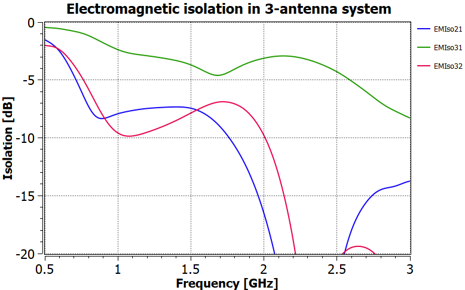# Optenni Lab: Calculation of electromagnetic isolation

#### When we consider the isolation between a pair of antennas, the result depends upon the impedance mismatch of each antenna. This means that an apparently good isolation may simply be due to mismatch, and when the antennas have properly been matched, the isolation can actually be very poor.EM isolation of a 3-antenna system: antenna 2 can be paired with either of the antennas 1 or 3 to form a MIMO system at 2.4 GHz, but antennas 1 and 3 must operate at different bands.

Optenni Lab provides a so called electromagnetic isolation analysis where this effect is factored out, and the isolation is calculated as if both ports were perfectly matched. If there are more than two antennas in the system, the electromagnetic isolation is calculated for each antenna pair with the assumption that the other ports are either left open or terminated at their impedances (typically 50 Ohms, but the termination impedance can also be different and frequency-dependent).

Electromagnetic isolation calculation allows you for example to

• Study the effect of antenna placement to isolation – this works great also in a 2-port measurement, as the calculation of electromagnetic isolation is carried out essentially real time with the VNA measurement
• Design a proper band allocation in a multi-antenna system such that the coupling is minimized